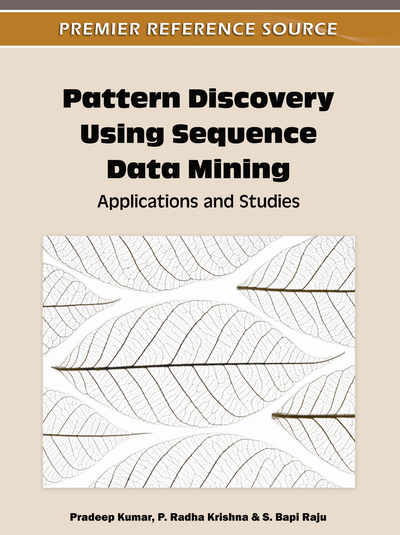# Mining Statistically Significant Substrings Based on the Chi-Square Measure

Sourav Dutta (IBM Research Lab, India) and Arnab Bhattacharya (Indian Institute of Technology Kanpur, India)
DOI: 10.4018/978-1-61350-056-9.ch004

## Abstract

With the tremendous expansion of reservoirs of sequence data stored worldwide, efficient mining of large string databases in various domains including intrusion detection systems, player statistics, texts, and proteins, has emerged as a practical challenge. Searching for an unusual pattern within long strings of data is one of the foremost requirements for many diverse applications. Given a string, the problem is to identify the substrings that differ the most from the expected or normal behavior, i.e., the substrings that are statistically significant (or, in other words, less likely to occur due to chance alone). We first survey and analyze the different statistical measures available to meet this end. Next, we argue that the most appropriate metric is the chi-square measure. Finally, we discuss different approaches and algorithms proposed for retrieving the top-k substrings with the largest chi-square measure.
Chapter Preview
Top

## Statistical Models And Tools

Establishing a relationship of the empirical or observed results of an experiment to factors affecting the system or to pure chance calls for various statistical models and measures. In such scenarios, an observation is deemed statistically significant if its presence cannot be attributed to randomness alone. The literature hosts a number of statistical models to capture the uniqueness of such observations such as p-value and z-score. In the next few sections, we discuss different important statistical tools that are used for this purpose.

Before venturing forward, we provide a formal definition of the problem.

Problem 1. Given a string S of length l comprising symbols from the alphabet set Σ of cardinality m, and with a given probability distribution P modeling the chance of occurrence of each symbol in Σ, the problem is to efficiently identify and extract the top-k substrings that exhibit the largest deviation from the expected nature, i.e., the substrings that are most statistically significant.

It is this measure of deviation of a sequence that we will capture by using various statistical models. In the remainder of the chapter, we interchangeably use the term string with sequence and substring with subsequence.

### Hypothesis Testing and P-value

Given an observation sample X (in this case a substring), with an associated score of S(X), the p-value of X is defined as the probability of obtaining a random sample with score S(X) or greater under the same probability model (Bejerano et al., 2004; Regnier & Vandenbogaert, 2006). For each such observation, we test the null hypothesis H0 that the substring is drawn from the given probability model P against the alternate hypothesis H1 that the subsequence is not drawn from the same probability distribution. The p-value measures the chance of rejecting the null hypothesis; in other words, the less the p-value, the less likely it is that the null hypothesis is true.

Figure 1 shows an example. For a particular score S, the shaded area represents the chance of having a sample with a score greater than the one under consideration. In other words, the p-value is the value of the cumulative density function (cdf) measured at S subtracted from the total probability, i.e.,

pvalue(S)=1-cdf(S).

If the probability density function (pdf) of the scores is known, it is relatively simpler to compute the p-value of a particular score using the above formula. However, in most real situations, the pdf is hard to estimate or can be non-parametric. The accurate computation of the p-value then needs all the possible outcomes to be listed, their scores computed, and the number of outcomes having scores more than S counted. Since the number of possible outcomes is large, and is exponential in most cases, computing the p-value in such a manner is practically infeasible.

To alleviate this problem, various branch-and-bound techniques have been proposed (Bejerano et al., 2004). In systems where such accuracy in measurement is not a necessity and a small factor of error can be tolerated, an approximation of the p-value can be calculated using other statistical tools (Rahmann, 2003).

## Complete Chapter List

Search this Book:
Reset